×#### Thank you for registering.

One of our academic counsellors will contact you within 1 working day.

Click to Chat

1800-1023-196

+91-120-4616500

CART 0

• 0

MY CART (5)

Use Coupon: CART20 and get 20% off on all online Study Material

ITEM
DETAILS
MRP
DISCOUNT
FINAL PRICE
Total Price: Rs.

There are no items in this cart.
Continue Shopping• Complete JEE Main/Advanced Course and Test Series
• OFFERED PRICE: Rs. 15,900
• View Details

```Chapter 32: Statistics – Exercise 32.7

Statistics – Exercise – 32.7 – Q.1

We observe that the average monthly wages in both firms is same i.e. Rs. 2500. Therefore the plant with greater variance will have greater variability.

Thus plant B has greater variability in individual wages.

Statistics – Exercise – 32.7 – Q.2

We observe that the average weights and heights for the 50 students is same i.e. 63.2.

Therefore, the parameter with greater variance will have more variability.

Thus, height has greater variability than weights.

Statistics – Exercise – 32.7 – Q.3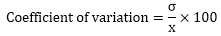So, we have:Statistics – Exercise – 32.7 – Q.4

CI
f
x
u = (x – A)/h
fu
u2
fu2

1000 – 1700
12
1350
-2
-24
4
48

1700 – 2400
18
2050
-1
-18
1
18

2400 – 3100
20
2750
0
0
0
0

3100 – 3800
25
3450
1
25
1
25

3800 – 4500
35
4150
2
70
4
140

4500 – 5200
10
4850
3
30
9
90

120

83

321

Here,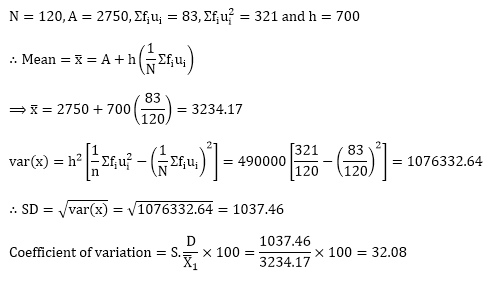Statistics – Exercise – 32.7 – Q.5

(i) Total wages paid by firm A = (Average wages) × (Number of employees)

= 52.5 × 587 = Rs 30817.50

Total wages paid by firm B = (Average wages) × (Number of employees)

= 47.5 × 648 = Rs 30780

So, firm A pays higher total wages,

(ii) In order to compare the variability of wages among the two firms, we have to calculated their coefficients of variation.

Let σ1 and σ2 denote the standard deviations of Firm A and Firm B respectively. Further,

let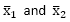be the mean wages in firms A and B respectively.

We have,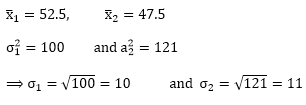Now,

Coefficient of variation in wages in firmand,

Coefficient of variation in wages in firmClearly, coefficient of variation in wages in greater for firm B than for firm A.

So, firm B shows more variability in wages.

Statistics – Exercise – 32.7 – Q.6

In order to compare the variability of weight in boys and girls, we have to calculate their coefficients of variation.

Let σ1 and σ2 denote the standard deviations of weight in boys and girls respectively. Further,

letbe the mean weight of boys and girls respectively.

we have,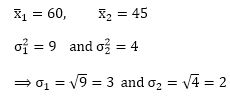Now,

Coefficient of variation in weights in boysand,

Coefficient of variation in weights in girlsClearly, Coefficient of variation in weights is greater in boys than in girls.

So, weights shows move variability in boys.

Statistics – Exercise – 32.7 – Q.7

In order to compare the variability of marks in Math, Physics, and Chemistry, we have to calculate their coefficients of variation.

Let σ1, σ2 and σ3 denote the standard deviations of marks in Math, Physics and chemistry respectively.

Further, let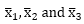be the mean scores in Math, Physics and Chemistry respectively.

We have,Now,

Coefficient of variation in MathsCoefficient of variation in Physics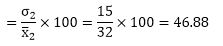Coefficient of variation in ChemistryClearly, Coefficient of variation in marks is greatest in Chemistry and lowest in Math.

So, marks in chemistry show highest variability and marks in maths show lowest variability.

Statistics – Exercise – 32.7 – Q.8

Let's first find the cofficient of variable for Group G1

CI
f
x
u = (x - A)/h
fu
u2
fu2

10 – 20
9
15
-3
- 27
9
81

20 – 30
17
25
-2
- 34
4
68

30 – 40
32
35
-1
- 32
1
32

40 – 50
33
45
0
0
0
0

50 – 60
40
55
1
40
1
40

60 – 70
10
65
2
20
4
40

70 – 80
9
75
3
27
9
81

150

-6

342

Here,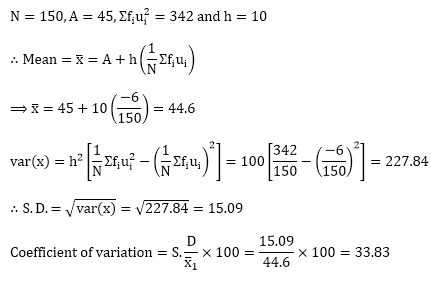Now, let's find the coefficient of variable for Group G2

CI
f
x
u = (x – A)/h
fu
u2
fu2

10 – 20
10
15
-3
30
9
90

20 – 30
20
25
-2
40
4
80

30 – 40
30
35
-1
30
1
30

40 – 50
25
45
0
0
0
0

50 – 60
43
55
1
43
1
43

60 – 70
15
65
2
30
4
60

70 – 80
7
75
3
21
9
63

150

-6

366

Here,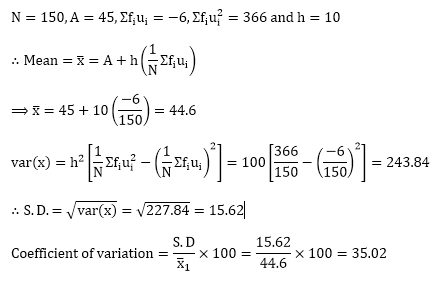∴  Group G2 is more variable.

Statistics – Exercise – 32.7 – Q.9

CI
f
x
u = (x - A)/h
fu
u2
fu2

10 – 15
2
12.5
-2
-4
4
8

15 – 20
8
17.5
-1
-8
1
8

20 – 25
20
22.5
0
0
0
0

25 – 30
35
27.5
1
35
1
35

30 – 35
20
32.5
2
40
4
80

35 – 40
15
37.5
3
45
9
135

100

108

266

Here,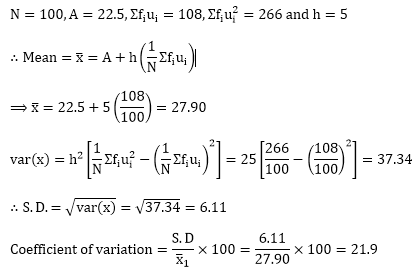Statistics – Exercise – 32.7 – Q.10

x
d = (x – Mean)
d2

35
-13
169

24
-24
576

52
4
16

53
5
25

56
8
64

58
10
100

52
4
16

50
2
4

51
3
9

49
1
1

480

980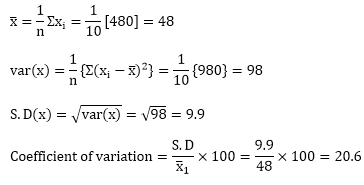x
d = (x – Mean)
d2

35
-13
169

24
-24
576

52
4
16

53
5
25

56
8
64

58
10
100

52
4
16

50
2
4

51
3
9

49
1
1

480

980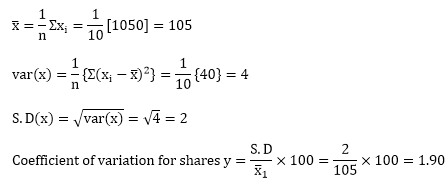Since the coefficient of variation for shares Y is smaller than the coefficient of variation for shares X, they are more stable.
```### Course Features

• 728 Video Lectures
• Revision Notes
• Previous Year Papers
• Mind Map
• Study Planner
• NCERT Solutions
• Discussion Forum
• Test paper with Video Solution